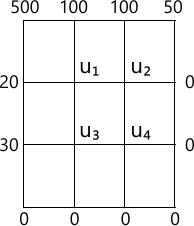MORE IN Engineering Mathematics 3
VTU Computer Science (Semester 3)
Engineering Mathematics 3
December 2013
Total marks: --
Total time: --
INSTRUCTIONS
(1) Assume appropriate data and state your reasons
(2) Marks are given to the right of every question
(3) Draw neat diagrams wherever necessary

1 (a) Find the Fourier series expansion of the function f(x) = |x| in (-? ?), hence deduce that $\dfrac {\pi^2}{8}=\sum ^{\infty}_{n=1}\dfrac {1}{(2n-1)^2}$
6 M
1 (b) Obtain the half range cosin series for the function, f(x)=(x-1)2 in the interval 0 ? x ? 1 and hence show that $\pi^2 =8 \left \{ \dfrac {1}{1^2}+\dfrac {1}{3^2}+\dfrac {1}{5^2} +......\right \}$
7 M
1 (c) Compute the constant term and first two harmonics of the Fourier series of f(x) given by.
$\begin{array}{|c|c|c|} \hline\text{x} & 0& \dfrac {\pi}{3} & \frac {2\pi}{3} & \pi & \frac {4\pi} {3} & \frac {5\pi}{3} & 2\pi \\ \hline \text{f(x)} & 1.0 & 1.4&1.9&1.7&1.5&1.2&1.0 \\ \hline \end{array}$
7 M

2 (a) Open the Fourier cosine transform of $f(x)=\dfrac {1} {1+x^2}$
6 M
2 (b) Find the Fourier transform of $f(x)=\left\{\begin{matrix} 1-x^2&for &|x|\le 1 \\0 &for &|x|>1 \end{matrix}\right. \ and \ evaluate \ \int_{n}\dfrac {x \cos x - \sin x}{x^3}dx$
7 M
2 (c) Find the inverse Fourier transform of $\dfrac {s}{1+s^2}$
7 M

3 (a) Obtain the various possible solutions of two dimensional Laplace's equation, uxx + uyy=0 by the method of separation of variables,
7 M
3 (b) Solve the one dimensional wave equation. $C^2 \dfrac {\partial^2 u}{\partial x}=\dfrac {\partial^2 u}{\partial t^2}, 0 < x0 is constant \[ (iii) \ \dfrac {\partial u}{\partial t}(x,0)=0$
7 M
3 (c) Obtain the D' Almbert's solution of the wave equation un=C2uxx subject to the conditions u(x,0)=f(x) and $\dfrac {\partial u}{\partial t}(x,0)=0$
6 M

4 (a) Find the best values of a, b, c, if the equation y=a+bx+cx2 is to fit most closely to the following observations.
 x 1 2 3 4 5 y 10 12 13 16 19
7 M
4 (b) Solve the following by graphical method to maximize z=50x+60y subject to the constraints. 2x+3y? 1500, 3x+2y?1500, 0? x ? 400 and 0 ? y ? 400.
6 M
4 (c) By using Simplex method. Maximize P= 4x1-2x2-x3 subject to the constraints.
x1+x2+x3?3, 2x1+2x2+x3?4, x1-x2?0, x1 ? 0 and x2 ? 0
7 M

5 (a) Using Newton-Raphson method find a real root of x sin x + cos x =0 nearer to ? carryout three iterations upto 4-decimal places.
7 M
5 (b) Find the largest eigen value and the corresponding eigen vector of the matrix.
$\begin{bmatrix} 2&-1 &0 \\-1 &2 &-1 \\0 & -1 &2 \end{bmatrix}$ BY using the power method by taking the initial vector as [1 1 1]T carryout 5-iterations.
7 M
5 (c) Solve the following system of equations by relaxation method:
12x+y+z=31, 2x+8y-z=24, 3x+4y+10z=58
6 M

6 (a) A survey conducted in a slum locality reveals the following information as classified below.
7 M
6 (b) Determine f(x) as a polynomials in x for the data given below by using the Newton's divided difference formula.
 x 2 4 5 6 8 10 f(x) 10 96 196 350 868 1746
7 M
6 (c) $Evaluate \ \int^1_0\dfrac {x}{1+x^2}dx \ by \ using \ Simpson's \ \left ( \dfrac {1}{3} \right )^{rd}$ rule by taking 6-equal strips and hence deduce an approximate value of log e2.
6 M

7 (a) Solve the wave equation, $\dfrac {\partial^2 u}{\partial t^2}=4\dfrac {\partial^2 u}{\parial x^2}$ subjects to u(0, 1)=0, u(4, t)=0, ut(x,0)=0 and u(x,0)=x(4-x) by taking h=1, K=0.5 upto 4-steps.
7 M
7 (b) Solve numerically the equation $\dfrac {\partial u}{\partial t}=\dfrac {\partial^2u}{\partial x^2}$ subject to the conditions u(0,t)=0=u(1,t),t?0 and u(x,0)=sin ?x, 0 ? x ? 1. Carryout computations for two levels taking h=1/3 and k=1/36.
7 M
7 (c) Solve uxx+uyy=0 in the following square region with the boundary conditions as indicated in the fig. Q7(c)6 M

8 (a) Find the z-transform of (i) sinh n? (ii) cosh n? (iii) n2
7 M
8 (b) Find the inverse z-transform of $\dfrac {2z^3+3z}{(z+2)(z-4)}$
6 M
8 (c) Solve the difference equation yn+2 +6yn+1+9yn=2n with y0=y1=0 using Z transforms.
7 M

More question papers from Engineering Mathematics 3